# PSAT Math : Basic Squaring / Square Roots

## Example Questions

← Previous 1 3 4 5

### Example Question #1 : How To Divide Square Roots

Divide and simplify. Assume all integers are positive real numbers.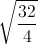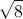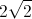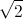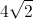Explanation:There are two ways to solve this problem. First you can divide the numbers under the radical. Then simplify.

Example 1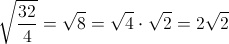Example 2

Find the square root of both numerator and denominator, simplifying as much as possible then dividing out like terms.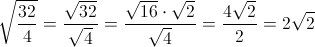Both methods will give you the correct answer of.

### Example Question #1 : How To Divide Square Roots

(√27 + √12) / √3 is equal to

√3

5/√3

(6√3)/√3

5

18

5

Explanation:

√27 is the same as 3√3, while √12 is the same as 2√3.

3√3 + 2√3 = 5√3

(5√3)/(√3) = 5

### Example Question #2 : How To Divide Square Roots

Simplify: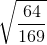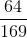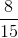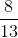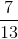Explanation:

To simplfy, we must first distribute the square root.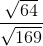Next, we can simplify each of the square roots.### Example Question #3 : How To Divide Square Roots

Find the quotient: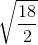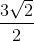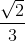Explanation:

Find the quotient: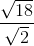There are two ways to approach this problem.

Option 1: Combine the radicals first, the reduce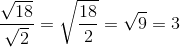Option 2: Simplify the radicals first, then reduce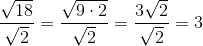### Example Question #4 : How To Divide Square Roots

Find the quotient: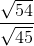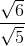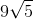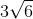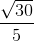Explanation: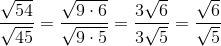Rationalize the denominator: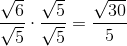### Example Question #21 : Basic Squaring / Square Roots

Evaluate: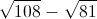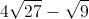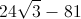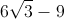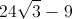Explanation:

Let us factor 108 and 81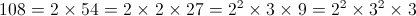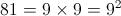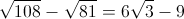### Example Question #1 : How To Subtract Square Roots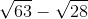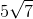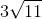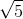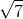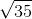Explanation:Step one: Find the greatest square factor of each radical

Forthis is, and forit is.

Therefore: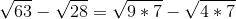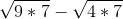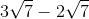### Example Question #1 : How To Add Square Roots

Simplify.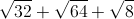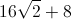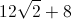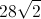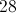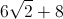Explanation:First step is to find perfect squares in all of our radicans.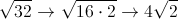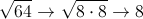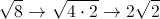After doing so you are left with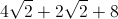*Just like fractions you can only add together coefficents with like terms under the radical. *### Example Question #1 : How To Add Square Roots

If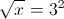what is?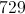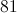Explanation:

Square both sides:

x = (32)2 = 92 = 81

### Example Question #2 : How To Add Square Roots

Simplify: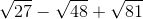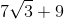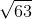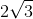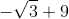Explanation:

To combine radicals, they must have the same radicand. Therefore, we must find the perfect squares in each of our square roots and pull them out.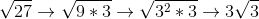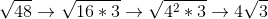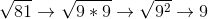Now, we plug these equivalent expressions back into our equation and simplify: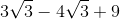← Previous 1 3 4 5